Latest SSC jobs   »   Govt Jobs 2022   »   Average Formula

# Study Notes On Average Formula With Examples

## Average Formula

Average can be defined as the mean of a certain set of numbers. Questions based on average are seen in various competitive exams, especially government job examinations. Candidates must be aware of the basic concepts, formulas, and methods required to solve the questions on Average. We are providing you with the study notes on Average along with Average Formulas and Examples.

## What is Average?

In maths, Average is the calculated “central” value of a set of numbers. The term ‘average’ refers to the ‘middle’ or ‘central’ point. In simple words, Average refers to a number that is a typical representation of a set of data. In Mathematics, Average is defined as the mean value which is equal to the ratio of the sum of all the data to the total no. of values/units present in the set. For example, the average of 3, 6, and 9 is 2 + 7 + 9 = 18÷ 3 = 6. So the average is 6. It means 6 is the central value of 3, 6, and 9. Therefore, average means to find out the mean value of a group of numbers.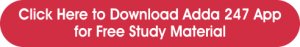## Average Symbol

• We can define average as the mean of the values which are represented by x̄ (x bar) also known as the average symbol.

• The average symbol also denoted by ‘μ’.

## Average Formula & Tricks

Average= (Sum of quantities)/(Number of quantities)

Having n number of values such as x1, x2, x3 ,….., xn.

So, the average or the mean of the given data will be equal to: Average = (x1+x2+x3+…+xn)/

• Average of two or more groups taken together

(a) If the number of quantities in two groups be n₁ and n₂ and their average is x and y, respectively, the combined average (average of all of them put together) is /

(n₁ x+n₂ y)/(n₁+n₂ )

(b) If the average of n₁ quantities is x and the average of n₂ quantities out of them is y, the average of the remaining group (rest of the quantities) is –

(n₁ x-n₂ y)/(n₁ – n₂ )

## What is Average Speed?

Average Speed is the rate at which a journey takes place. Throughout a journey, the speed is not constant, it varies from time to time. Average Speed helps to give an estimate of the rate at which the journey is completed. Let’s find out more about Average Speed.

## Average Speed Formula

The average speed of an object is equal to the total distance covered by the object, divided by the total time taken to cover the distance. When ‘D’ is the distance traveled in some time ‘T’ and the speed of the object for this journey or ‘s’ is equal to

Average Speed = Total distance covered ÷ Total time taken

s = D/T.

### Average Speed Formula Examples

Example 1: A car travels at a speed of  45 km/hr for 5 hours and then decides to slow down to 40 km/hr for the next 2 hours. Calculate the average speed of the car using the average speed formula.

Solution:

Distance I = 45 × 5 = 225 miles
Distance II = 40 × 2 = 80 miles
Total distance = Distance 1 + Distance 2
D = 225 + 80 = 305 miles
Using average speed formula = Total distance traveled ÷ Total time taken
Average Speed = 305 ÷ 7 = 43.57 m/s.

Answer: Average speed of a car is 43.57 m/s.

Example 2: A train is moving with a speed of 80 miles per hour for the first 4 hours and 110 miles per hour for the next 3 hours. Find the average speed of the train using the average speed formula.

Solution:

It is given that the train is moving at a speed of 80 miles per hour for the first 4 hours.
Here  = 80 and  = 4.
And the train is moving at a speed of 110 miles per hour for the next 3 hours.
Hence  = 110 and  = 3.
Average Speed Formula =
Average Speed = (80 × 4 + 110 × 3) ÷ (4 + 3)
= (650) ÷ (7) = 92.86 miles/hour

Example 3: With the help of the average speed formula, find the average speed of John, who covers the first 200 kilometers in 4 hours and the next 160 kilometers in another 4 hours.

Solution:

To find the average speed we need the total distance and the total time.
Total distance covered by Sam = 200Km + 160 km = 360 km
Total time taken by Sam = 4 hour + 4 hour = 8 hour
Average Speed = Total distance covered ÷ Total time taken
Average Speed = 360 ÷ 8 = 45km/hr

Answer: Average speed of John is 45 km/hr.

## What is Average Velocity?

Finding out the average value of a given number of velocities is known as average velocity. Average velocity is defined as the displacement of an object over time.

Average velocity is the difference between the starting and ending positions, divided by the difference between the starting and ending time taken.

• Velocity has a magnitude (a value) and a direction.
• The unit of velocity is meters per second (m/s).

## Average Velocity Formula

For Example, Average Velocity is the ratio of the displacement from point A to point B of an object to the time it takes to make that displacement from point A to point B.

Average Velocity = displacement / time

Average Velocity = (End positin – Start position) / (End Time) – (Start time)

= x2 – x1 / t2 – t1

where,
x1 = the start position of an object (m)
x2 = the end position of an object (m)
t1 = the start time of the motion (s)
t2 = the end time of the motion(s)

#### Click Here For Study Notes on Quantitative Aptitude For SSC CGL

Q. The average weight of 24 students of section A of a class is 58 kg whereas the average weight of 26 students of section B of the same class is 60.5 kg. Find the average weight of all the 50 students of the class.

Sol. Here n₁ = 24, n₂ = 26, x = 58 and y = 60.5.
∴ Average weight of all the 50 students
=(n₁ x+n₂ y)/(n₁+n₂ )
=(24×58+24×60.5)/(24+26)
=(1392+1573)/50=2965/50
= 59.3 kg

• The average of n quantities is equal to x. If one of the given quantities whose value is p, is replaced by a new quantity having value q, the average becomes y,  then
q = p + n(y – x)

Q. The average weight of 25 persons is increased by 2 kg when one of them whose weight is 60 kg is replaced by a new person. What is the weight of the new person?

Sol. The weight of the new person
= p + n(y – x)
= 60 + 25(2) = 110 kg

• The average of n quantities is equal to x. When a quantity is removed, the average becomes y. The value of the removed quantity is
n(x – y) + y.
• The average of n quantities is equal to y. When a quantity is added, the average will become y. The value of the new quantity is
n(y – x) + y.

Q. The average age of 24 students and the class teacher is 16 years. If the class teacher’s age is excluded, the average age reduces by 1 year. What is the age of the class teacher?

Sol. The age of class teacher
= n (x – y) + y
= 25 (16 – 15) + 15
= 40 years

• The average of first n natural numbers is
(n + 1)/2.
• The average of square of natural numbers till n is
((n + 1)  (2n + 1))/6
• The average of cubes of natural numbers till n is
(n (n + 1)²)/4.
• The average of odd numbers from 1 to n is
(last odd number+1)/2.
• The average of even numbers from 1 to n is
(last even number + 2)/2.

#### Click Here to Attempt More Questions From Quantitative Aptitude For SSC CGL

Q. What is the average of odd numbers from 1 to 40?

Sol. The required average
=(last odd number+1)/2
=(39+1)/2
= 20

Q. What is the average of even numbers from 1 to 81?

Sol. The required average
=(last even number+2)/2
=(80+2)/2
= 41

• If n is odd: The average of n consecutive numbers, consecutive even numbers or consecutive odd numbers is always the middle number.
• If n is even: The average of n consecutive numbers, consecutive even numbers or consecutive odd numbers is always the average of the middle two numbers.
• The average of first n consecutive even numbers is   (n + 1)
• The average of first n consecutive odd numbers is n.
•  The average of squares of first n consecutive even numbers is
(2 (n + 1)  (2n + 1))/3.
• The average of squares of consecutive even numbers till n is
((n + 1)  (n + 2))/3.
• The average of squares of consecutive odd numbers till n is
(n (n + 2))/3.
• If the average of n consecutive number is m, then the difference between the smallest and the largest number if
2 (n – 1).

Q. Find the average of squares of first 19 consecutive even numbers.

Sol. The required average
=(2 (n+1)(2n+1))/3=(2(19+1)(2×19+1))/3
=(2×20×39)/3=1560/3=520

Q. Find the average of squares of consecutive odd numbers from 1 to 31.

Sol. The required average
=(n (n+2))/3=(31×(31+2))/3=(31×33)/3=341

Q Find the average velocity when the changes in displacement in three consecutive instances are 8 m, 10 m, 12 m, and the total time taken is 6 s.

Explanation: The total change in displacement = 30 m that is, (8+10+12). Now, the total time is taken = 6 s. Therefore, the Average velocity = total change in displacement/total time taken = 30/6 = 5 m/s.

### Average Formula- FAQs

Q. How do you calculate Average?

Ans: Average is calculated by adding a group of numbers and dividing that sum by the count of those numbers. For example, the average of 3, 4, 5, 6, 7, and 11 is 36 divided by 6 , which is 6.

Q. What are the three ways to calculate Average?

Ans: The three most common measures of central tendency are: mean, median and mode.

Q. What is the basic formula of Average?

Ans: The basic formula of Average is the sum of all the values divided by the total number of values.

Q. Why Average is calculated?

Ans:  Average is calculated to represent a large set of numbers with a single number. Average represents all the numbers available in the data set.

### Click here for SSC CGL Tier 2 Free Quizzes of all Topics

#### Congratulations!

General Awareness & Science Capsule PDF

Incorrect details? Fill the form again here

General Awareness & Science Capsule PDF

Thank You, Your details have been submitted we will get back to you.
•Time and Work Notes, Simple Tricks To So...
•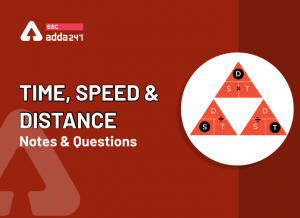Speed, Time And Distance Tricks and Ques...
•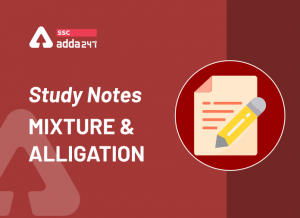Mixture and Alligation, Notes, Methods a...
•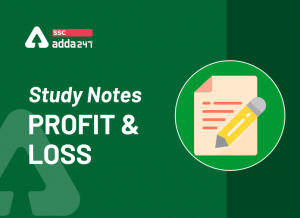Profit and Loss Formula, Concept and Stu...
•Target SSC CGL Tier 2: How to Score Full...
•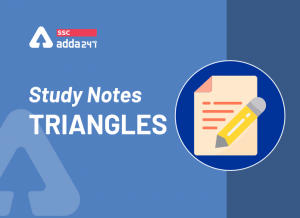Triangles: Notes and Questions